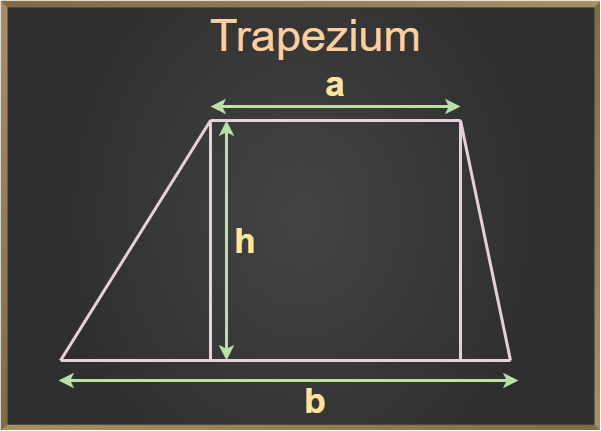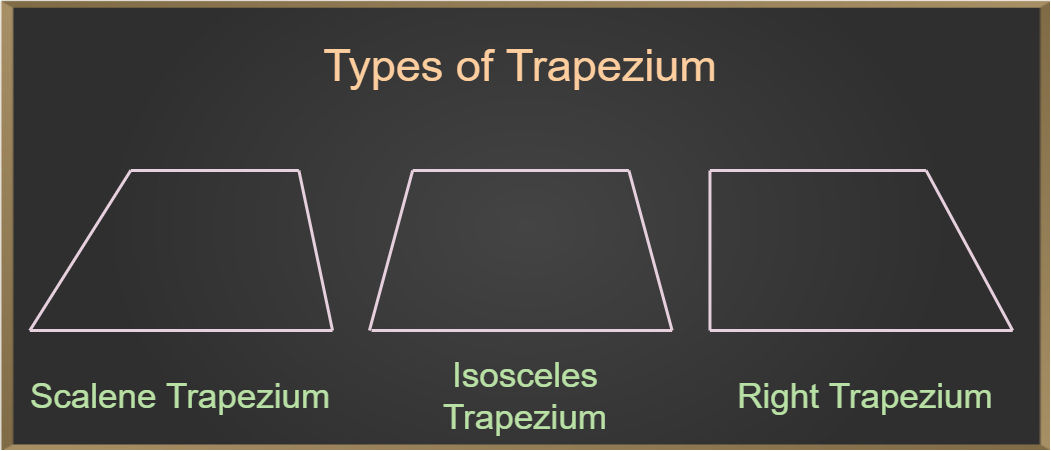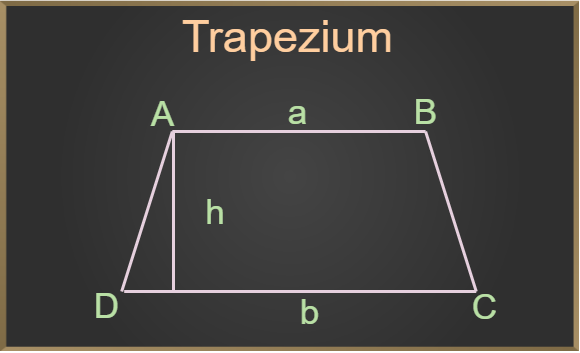Open in App
Not now

## Related Articles

• CBSE Class 8 Syllabus (All Subjects)
• CBSE Class 8 Maths Notes
• CBSE Class 8 Science Revision Notes
• CBSE Class 8 Social Science Revision Notes
• CBSE Class 8 History Revision Notes
• CBSE Class 8 Civics Revision Notes
• CBSE Class 8 Geography Revision Notes

# Trapezium

• Difficulty Level : Expert
• Last Updated : 09 Nov, 2022

The trapezium is a polygon with four sides, i.e. it is a quadrilateral. A trapezium is a special quadrilateral with only one pair of parallel sides. The trapezium is a two-dimensional shape that appears as a table. Trapezium originated from the Greek word “trapeze” which means table.

## What is a Trapezium?

A trapezium is a closed-shaped two-dimensional quadrilateral having a pair of parallel opposite sides. The parallel sides of a trapezium are called bases and the non-parallel sides of a trapezium are called legs. The trapezium has four sides and four corners. A parallelogram is also called a trapezoid with two parallel sides.In the above figure, a and b are the bases of the trapezium and h is the height of the trapezium.

## Types of Trapezium

Based on the sides and the angles, the trapezium is of three types:

• Scalene Trapezium
• Isosceles Trapezium
• Right Trapezium### Isosceles Trapezium

The trapezium which has an equal length of legs is called an isosceles trapezium, i.e. in an Isosceles Trapezium, the two non-parallel sides are equal.

### Scalene Trapezium

A trapezium with all the sides not equal is called a scalene trapezium. In a scalene trapezium, no two angles are equal.

### Right Trapezium

A trapezium that has a right angle pair, adjacent to each other is known as a right trapezium.

## Irregular Trapezium

A trapezium has one pair of parallel sides and the other two sides are non-parallel. In a regular trapezium, the other two non-parallel sides are equal, but in the case of an irregular trapezium the two non-parallel opposite sides, are unequal.

## Properties of Trapezium• In trapezium, bases are parallel to each other. Example – The sides AB and CD are parallel to each other, shown in the figure.
• The adjacent interior angles in a trapezium sum up to 180°. Example – There are two pairs of co-interior angles. One pair is ∠ A and ∠ D whereas the other pair is ∠ B and ∠ C. The sum of each pair of co-interior angles is 180°.
• The sum of all the interior angles in a trapezium is always 360°. Example – In the figure ∠A+∠D is 180°  and ∠B+∠C is 180°. Therefore ∠A+∠D +∠B+∠C = 360°.
• The length of both diagonals in the trapezium is equal.
• In the trapezium, both diagonals intersect each other.
• Trapezium has exactly one pair of opposite sides that are parallel.

## Trapezium Formulas

The important formulas of a trapezium are:

• Area of Trapezium =  ½ (Sum of parallel sides) × (Distance between parallel sides)
• Perimeter of Trapezium = Sum of all four sides

## Area of Trapezium

Trapezium has two parallel sides a, and b units respectively, and its altitude is h.

Now the area of the trapezium can be calculated by finding the average of bases and multiplying its result by the altitude.

Hence,

Area of trapezium  = ((a +b)/2) × h

where,
a and b are the bases of trapezium
h is the altitude

### Area of Isosceles Trapezium

Let a and b be the length of parallel sides of a trapezium ABCD, where a and b are the bases of the trapezium and a>b.

Now, as it is an Isosceles Trapezium c is the length of both the two non-parallel sides and h is the height of the trapezium.

Npw, AB = a, CD = b, BC = AD = c

In the right triangle, AED

Length of perpendicular, h = √(c2 – (a-b)2)   [using Pythagoras theorem]….(1)

Now,
Area = ½ × sum of parallel sides × height of trapezium
= ½ × (a+b) × h

using equation (1)

Area of Isosceles Trapezium = 1/2 × [√(c2 – (a-b)2) (a+b)]

## Perimeter of Trapezium

The perimeter of a trapezium is given by calculating the sum of all its sides.

Hence,

Perimeter of trapezium = AB + BC + CD + AD

where
AB, BC, CD and AD are the sides of the trapezium.

### Perimeter of Isosceles Trapezium

If in an Isosceles trapezium a and b are the lengths of parallel sides i.e. the bases and c is the length of two equal non-parallel sides, then the perimeter is given by:

Perimeter = a+b+2c

where
a, b are bases
c is the equal side

## Difference between Trapezium and Trapezoid

In general terms, both Trapezium and Trapezoid are the same but the difference lies in their country of origin.

The trapezium is of British origin, it is a four-sided polygon and a two-dimensional figure it has exactly one pair of parallel sides opposite to each other. In India, we follow British English hence, the word Trapezium is used.

The trapezoid is of American origin, it is also a four-sided polygon with one pair of parallel sides opposite to each other. Parallel sides are the bases and another two non-parallel sides are called the legs of the trapezoid.

## Solved Examples on Trapezium

Example 1: Find the fourth side of the trapezium, if the other three sides are 8 cm, 12 cm, and 16 cm, and the perimeter is 40 cm.

Solution:

Perimeter is given as the sum of all its sides. Let the length o unknown be ‘x’ units.

Perimeter =  40

40 = 8 + 12 + 16 + x

x = 40 – (8 + 12 + 16)

= 4 cm

Thus, the length of the unknown side is 4 cm

Example 2: A trapezium has parallel sides of lengths 15 cm and 11 cm, and non-parallel sides of length 5 cm each. Calculate the perimeter of the trapezium.

Solution:

It is a Isosceles Trapezium because it is clearly mentioned that non parallel sides of length 5 cm each are equal. According to the isosceles trapezium if two non-parallel sides of the trapezium are of equal length then it is known as isosceles trapezium.

Given, a=15 cm, b=11 cm and c= 5 cm

We get,

Perimeter = a+b+2c

P = 15+11+2(5)

P = 15+11+10

P = 36 cm

Example 3: Find the perimeter of a trapezium whose sides are 12cm, 14cm, 16cm, and 18cm.

Solution:

As we know that the perimeter of a trapezium is given by calculating the sum of all its sides.

P = Sum of all the sides

P = 12 + 14 + 16 + 18

P = 60 cm

Hence, the perimeter of trapezium is 60 cm.

Example 4: Find the area of the Trapezium, in which the sum of the parallel sides is 60 cm, and its height is 10 cm.

Solution:

Given, the sum of parallel sides 60 cm and the height, h =10 cm

We know that,

Area of a trapezium, A = 1/2 × Sum of parallel sides × distance between the parallel sides

By substituting the given values,

A =1/2×60×10

A = 30×10

A = 300 cm2.

Therefore, the area of Trapezium =300 cm2.

## FAQs on Trapezium

Question 1: How many parallel sides do a Trapezium have?

We know that trapezium, is a quadrilateral with one pair of parallel sides. Thus, a trapezium has a pair of parallel lines(sides).

Question 2: Can a trapezium be considered a Quadrilateral?

A has four sides, four vertices, and four angles. Hence it can be considered a quadrilateral,  sum of all four interior angles of a trapezium is 360 degrees.

Question 3: Can a square be called a trapezium?

A trapezium is a quadrilateral with only one pair of parallel sides and the other two sides are non-parallel. But in the case of a Square, it has two pairs of parallel sides hence, it can not be considered a Trapezium.

Question 4: Are the diagonals of a trapezium always equal?

The diagonals of a trapezium may not be equal. In the case of a Regular polygon, the diagonals are equal but this is not true in the case of an Irregular Polygon.

Question 5. What are the properties of a trapezium?

The 5 properties of a Trapezium are:

• In trapezium, bases are parallel to each other.
• A trapezium has supplementary adjacent angles.
• Only one pair of opposite sides are parallel.
• The sum of all the interior angles in a trapezium is always 360°.
• The line that joins the mid-point of the non-parallel sides is always parallel to the bases.

### Related Resources

My Personal Notes arrow_drop_up